# 红黑树的定义

1、每个结点或者是红的，或者是黑的。

2、根结点是黑的。

3、每一个叶结点（nil结点,即最后的空结点）是黑的。

4、如果一个结点是红的，则其孩子结点都是黑的。

5、对于任意结点，从该结点到其子孙叶结点（nil结点）的所有路径上包含相同数目的黑结点。

# 2-3树

2-3树满足二分搜索树的基本性质。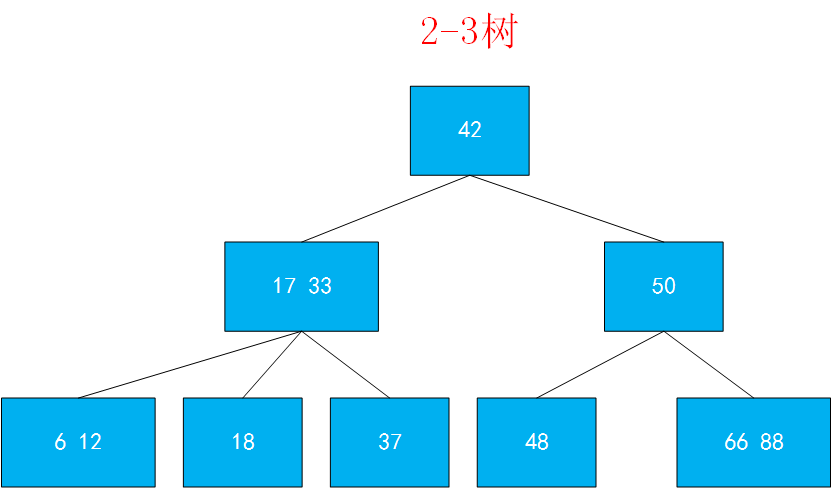2-3树是一棵绝对平衡的树。

## 2-3树的绝对平衡性

### 插入新键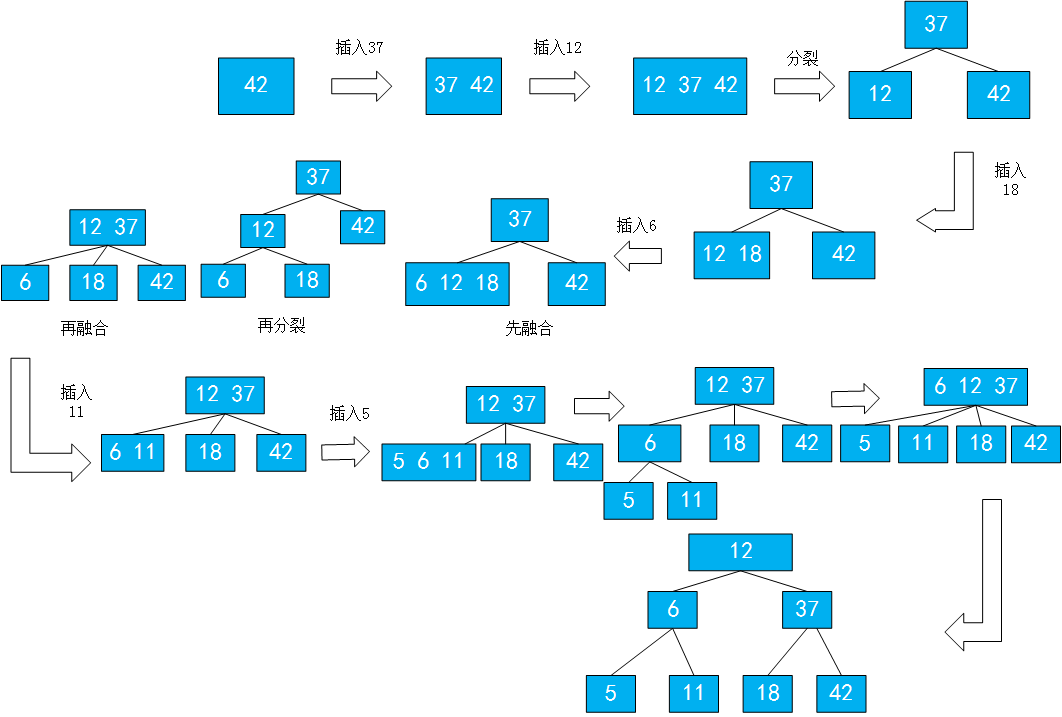# 2-3树和红黑树的等价性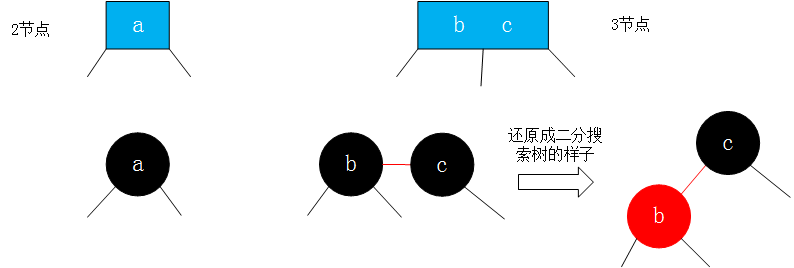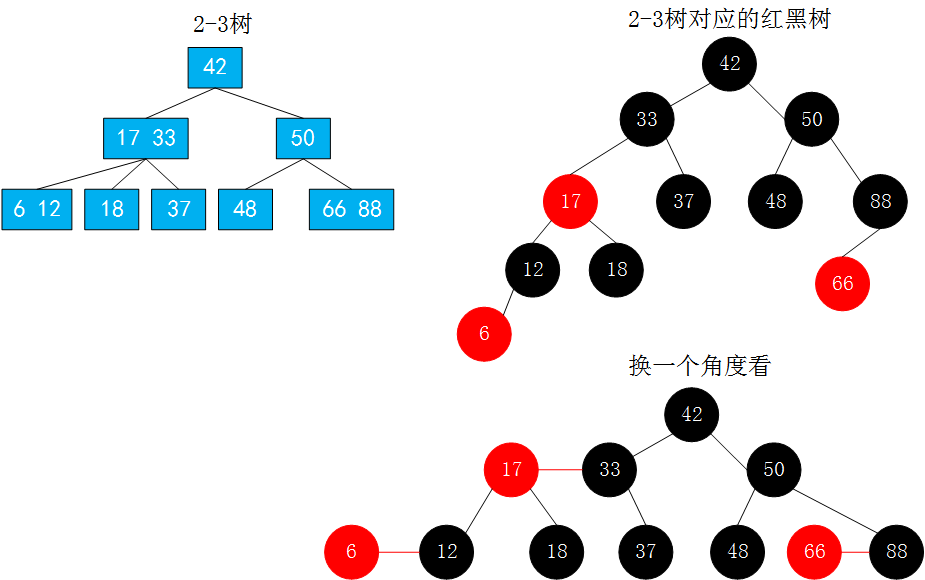# 颜色表示

``````import java.util.ArrayList;

public class RBTree<K extends Comparable<K>,V> {

private static final boolean RED = true;
private static final boolean BLACK = false;
private class Node{
public K key;
public V value;
public Node left,right;
public boolean color;//由其父结点指向它的链接的颜色

public Node(K key,V value){
this.key = key;
this.value = value;
left = null;
right = null;
color = RED; //初始化为红结点，相当于刚创建时就与其父结点进行融合，融合后的操作是后续的事。
}
}

private Node root;
private int size;

public RBTree() {
root = null;
size = 0;
}

// 返回以node为根节点的二分搜索树中，key所在的节点
private Node getNode(Node node, K key){

if(node == null)
return null;

if(key.equals(node.key))
return node;
else if(key.compareTo(node.key) < 0)
return getNode(node.left, key);
else // if(key.compareTo(node.key) > 0)
return getNode(node.right, key);
}

public boolean contains(K key){return getNode(root,key) != null;}

public V get(K key){
Node node = getNode(root,key);
return node == null ? null : node.value;
}
}
``````

# 在红黑树中添加新元素

1.最初情况是刚开始的树为空，然后让红黑树的根为新添加的元素，然后让根结点的红色变为黑色。

2.要添加的结点小于根结点，即添加到根结点的左孩子位置，则直接添加过去就可以了。

3.要添加的结点大于根结点，则先添加到根结点的右孩子位置，然后进行左旋转。左旋转方法和AVL树的左旋转类似。在左旋转后，有可能会产生两个红色结点(`x.color = node.color;node.color = RED;`)，这时候不用担心，因为返回的根结点如果为红色，它将会继续和上一层进行相关转换操作。

4.向红黑树中的3-结点添加元素时： ①：向3-结点的右孩子添加时，直接添加到右孩子，然后所有子结点变为黑结点，也就是相当于在2-3树中先将3-结点变为4-结点，然后再分离为3个2-结点，然后再和其上面的父结点进行融合，所以这里要将其根结点变为红色（这样才能保证在红黑树中这个结点和其父结点进行融合） ②：向3-结点添加元素时，新元素小于根结点的左孩子（即新键小于原树中的两个键），需要继续添加到根结点的左孩子的左孩子（即连接到最左边的空链接），它应该是一个红色结点，这样就产生了两个连续的红链接。此时需要将上层的红链接右旋转（中值键作为根结点并和其他两个结点用红链接相连） ③：如果新键的值介于原树中的两个树之间，这样又会产生两个连续的红链接，一条红色左链接一条红色右链接。这时候需要将下层的红链接左旋转得到第②种情况，然后在②情况的基础上继续操作。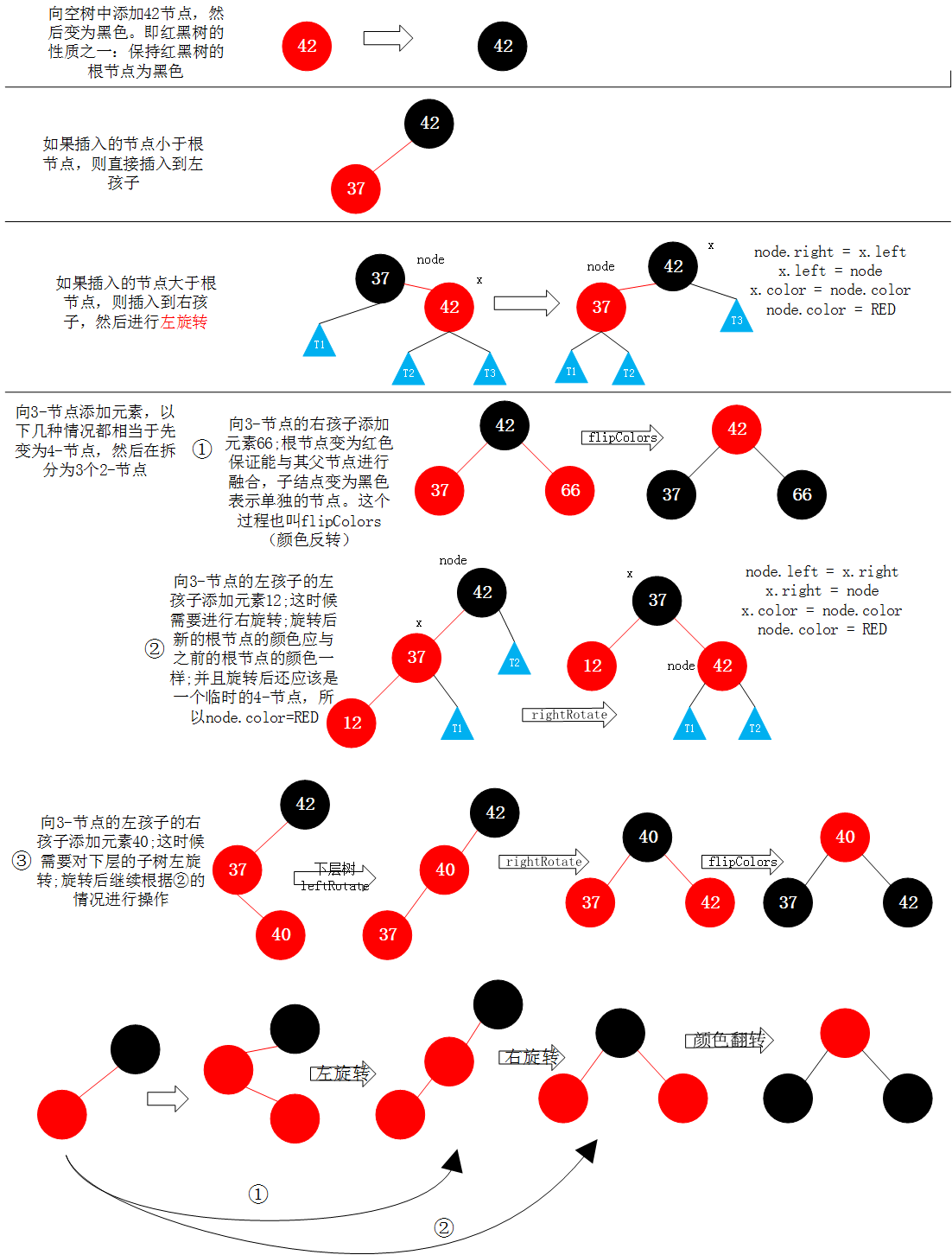``````
//判断节点node的颜色
private boolean isRed(Node node){
if(node ==null) {
return BLACK;
}
return node.color;
}

//左旋转
private Node leftRotate(Node node) {

Node x = node.right;

//左旋转操作
node.right = x.left;
x.left = node;

x.color = node.color;
node.color = RED;

return x;
}

//右旋转
private Node rightRotate(Node node) {
Node x = node.left;

//右旋转
node.left = x.right;
x.right = node;

x.color = node.color;
node.color = RED;

return x;
}

//颜色翻转
private void flipColors(Node node){

node.color = RED;
node.left.color = BLACK;
node.right.color = BLACK;
}

//向红黑树中添加新元素(key,value)
root.color = BLACK;//保证最后的根节点为黑色
}

//向以node为根的红黑树中插入元素(key,value),递归算法
//返回插入新节点后红黑树的根
private Node add(Node node,K key,V value){
if(node == null){
size ++;
return new Node(key,value); //默认插入红色结点
}

if(key.compareTo(node.key) < 0)
else if(key.compareTo(node.key) > 0)
else
node.value = value;

//插入结点结束后，进行红黑树性质的维护
//如果右孩子是红色，左孩子不是红色，则进行左旋转
if(isRed(node.right) && !isRed(node.left))
node = leftRotate(node);
//如果左孩子是红色，左孩子的左孩子也是红色，则进行右旋转
if(isRed(node.left) && isRed(node.left.left))
node = rightRotate(node);
//如果node的左孩子和右孩子都是红结点，则进行颜色反转
if(isRed(node.left) && isRed(node.right))
flipColors(node);

return node;
}
``````